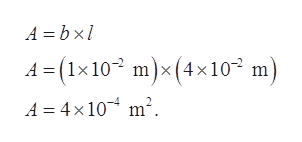# A thin 1.5-mm coating of glycerine has been placed between two microscope slides of width 1.0 cm and length 4.0 cm. Find the force required to pull one of the microscope slides at a constant speed of 0.30 m/s relative to the other slide.

Question
22 views

A thin 1.5-mm coating of glycerine has been placed between two microscope slides of width 1.0 cm and length 4.0 cm. Find the force required to pull one of the microscope slides at a constant speed of 0.30 m/s relative to the other slide.

check_circle

Step 1

Given:

Distance between two microscopic slides (d) = 1.5 mm = 1.5×10-3 m

Dynamic viscosity (η) = 1.5 Ns/m2.

Width of slides (b) = 1.0 cm

Length of slides (l) = 4.0 cm

Speed (v) = 0.3 m/s

Step 2

Area of slides is,help_outlineImage TranscriptioncloseA = bx1 1 = (1×10³ ×10² m) m)x(4 m². A = 4× 10 fullscreen
Step 3

Force required to pul...

### Want to see the full answer?

See Solution

#### Want to see this answer and more?

Solutions are written by subject experts who are available 24/7. Questions are typically answered within 1 hour.*

See Solution
*Response times may vary by subject and question.
Tagged in
SciencePhysics

### Fluid Mechanics